$\newcommand\si{\mathrm{#1}} \newcommand\SI{#1\,\si{#2}} \newcommand\matr{\mathbf{#1}} \DeclareMathOperator*{\argmax}{arg\,max} \DeclareMathOperator*{\argmin}{arg\,min}$

# Coordinate systems and data types in GIS

Institute for Environmental and Spatial Analysis...University of North Georgia

## 1   Datum

A datum describes the relationship between a spheroid model of the Earth and its real shape.

There are hundreds of datums and each one uses different methods to measure the Earth.

• WGS84 (World Geodetic System of 1984)
• NAD83 (North American Datum of 1983)
• NAD27 (North American Datum of 1927)

## 2   Geographic coordinate systems

A geographic coordinate system (GCS) uses a three-dimensional spherical surface.World Geodetic System of 1984 (WGS84)
\begin{align*} a&=\SI{6,378,137.0}{m}\\ b&=\SI{6,356,752.31424}{m}\\ \frac{1}{f}&=\frac{a}{a-b}=298.257223563 \end{align*}

• $a$: Semi-major axis
• $b$: Semi-minor axis
• $1/f$: Inverse flattening

## 3   Projected coordinate systemsCylindrical projection (e.g., Mercator projection)

### 3.1   Projection types

Secant conformal conic projection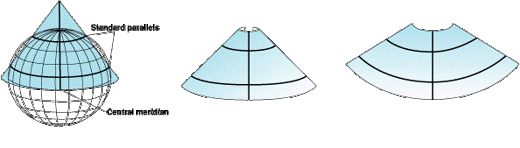Meridians are lines of longitude (vertical lines) that end at the North and South poles.

Parallels are circles of latitude (horizontal circles).

Tangent conformal conic projection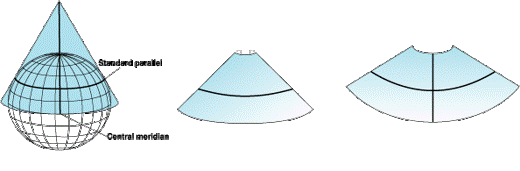## 4   Conformality vs. equivalency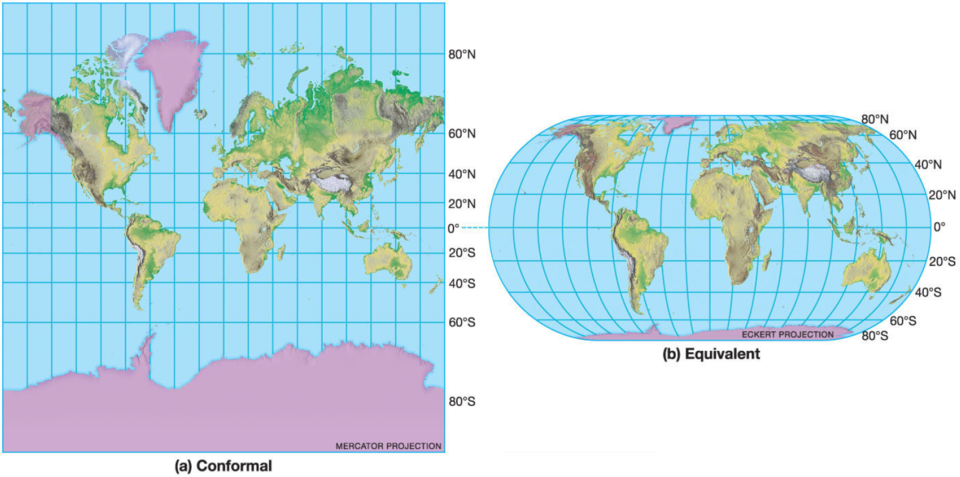Conformality

• Correct shape and angle (for smaller areas, e.g., coast lines)
• Incorrect size
• Conformal projections

Equivalency

• Correct size and area
• Incorrect shape
• Equal-area projections

## 5   Universal transverse Mercator (UTM)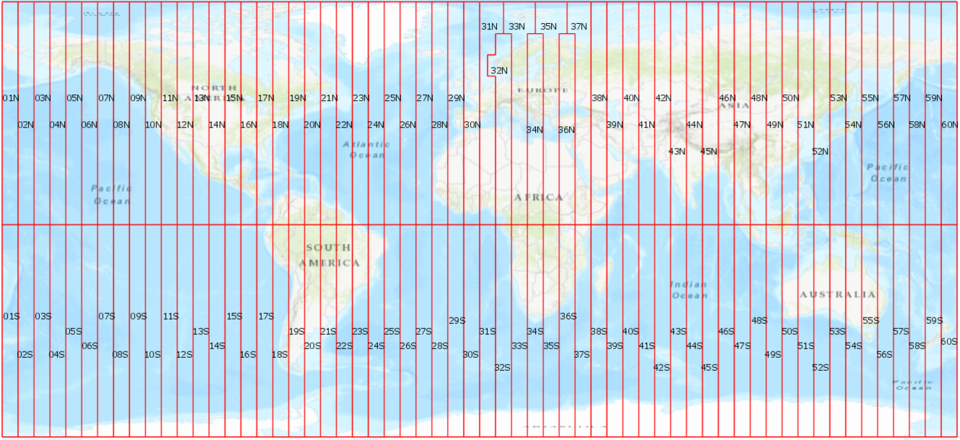• Each 6 degrees of longitude
• Large north-south extent with low distortion

## 6   State plane coordinate systemsConformal projections with an accuracy of one part in 10,000

## 7   Vector data

### 7.1   Points

Points are zero-dimensional objects and represent geographic features such as wells, sample locations, or trees.

### 7.2   Point data examples

• Soil samples
• Type
• PH
• Contaminants
• Utility poles
• Owner
• Height
• Attachments
• Spill locations
• Accident number
• Type of spill
• Extent
• Parcel centroids
• Section/block/lot no.
• Address
• Owner
• Assessment data

### 7.3   Point data: Light poles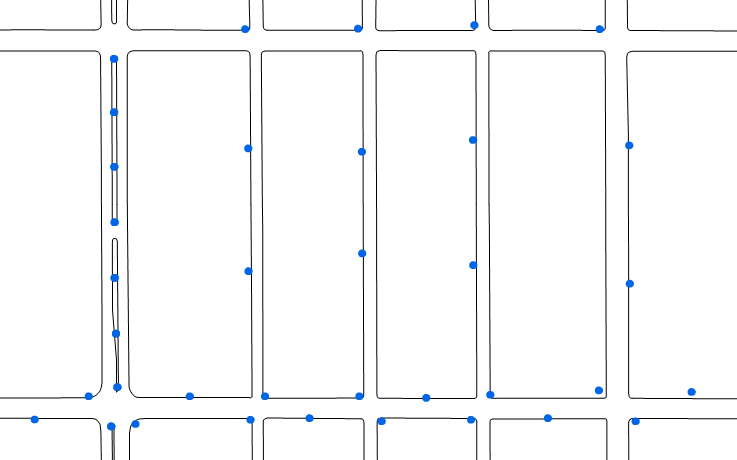### 7.4   Point data: Parcel centroids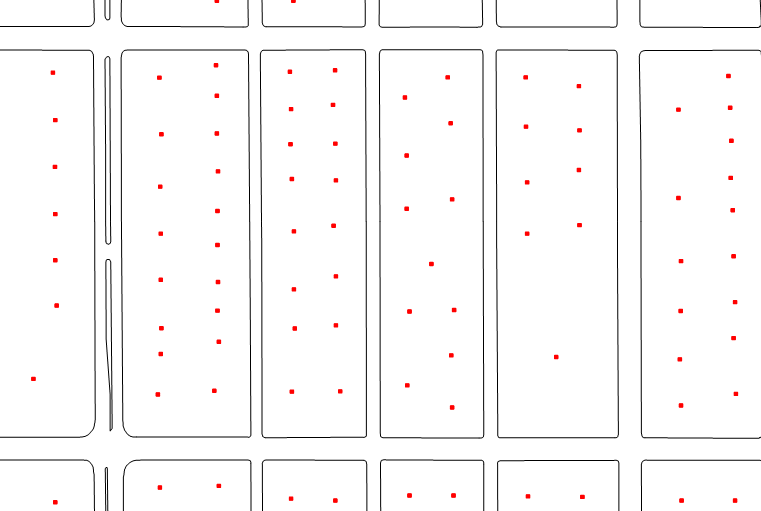### 7.5   Lines

Lines represent one-dimensional objects or linear features such as road and stream centerlines.

Lines are made up of a series of interconnected points.

A line typically starts and ends with a special point called a node, and the points that make up the rest of a line are called vertices.

### 7.6   Line data examples

• Street centerlines
• Street name
• Address ranges
• Water mains
• Pipe size
• Pipe material
• Date installed
• Streams
• Flow rate
• Cross-sectional area
• Depth
• Water quality

### 7.7   Line data: Street centerlines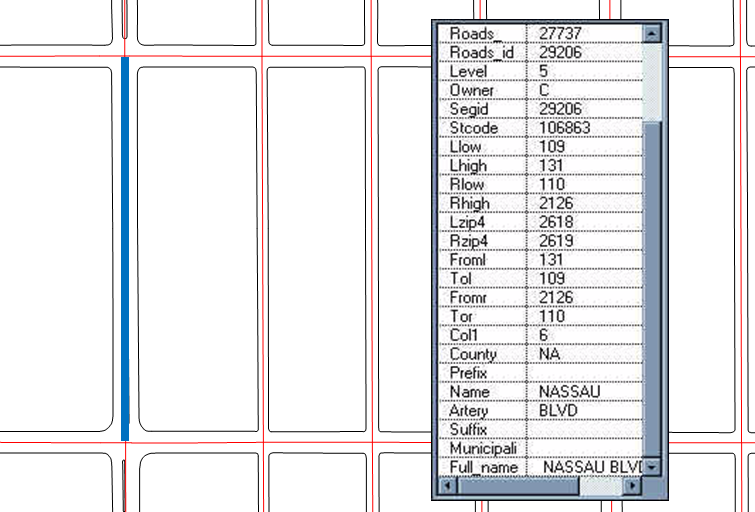### 7.8   Polygons

Polygons represent two-dimensional objects such as the boundaries of a field or property, or the outline of a building or lake.

Polygons are made up of a series of connected lines where the starting point of a polygon is the same as the ending point.

### 7.9   Polygon data examples

• Parcel
• Parcel ID
• Dimensions and area
• Soil boundaries
• Type
• Permeability
• Flood zones
• Flood depth
• Flood frequency

### 7.10   Polygon data: Parcels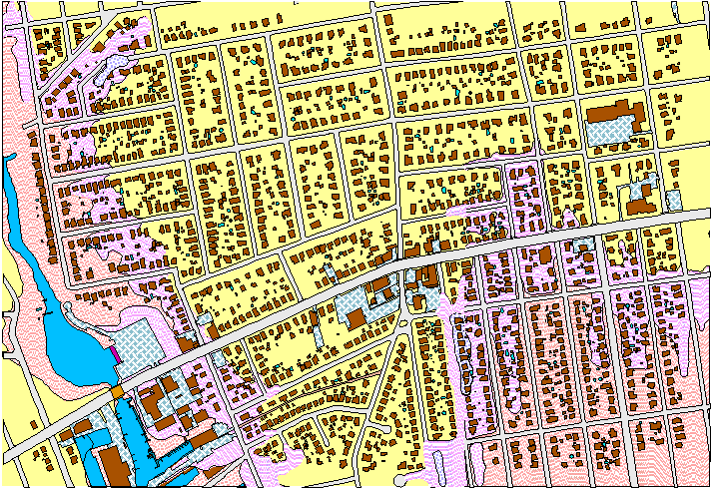### 7.11   Polygon data: Building footprints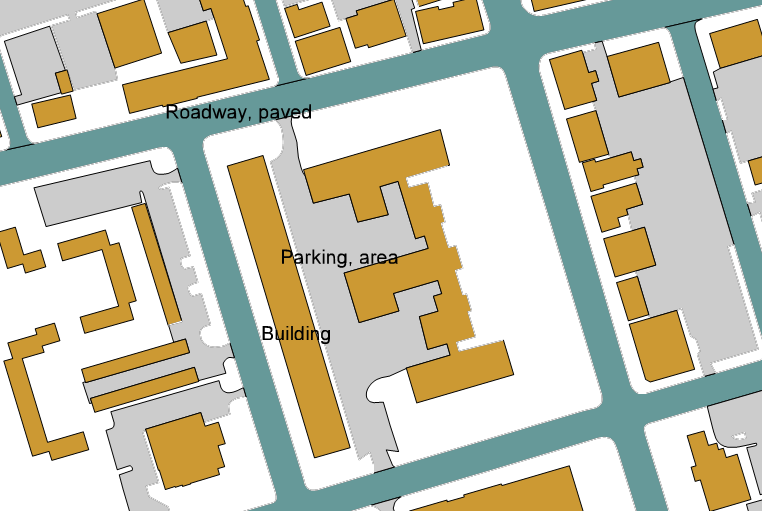## 8   Raster data

Geographic data sets

• Land use/land cover
• Vegetation index
• Soil stability

Digital photography

• Building photos
• Accident scenes
• Crop damages
• Full motion videos

Digital orthophotography

• Rectified aerial photos

### 8.1   Raster data structure

Matrix of equal-area cells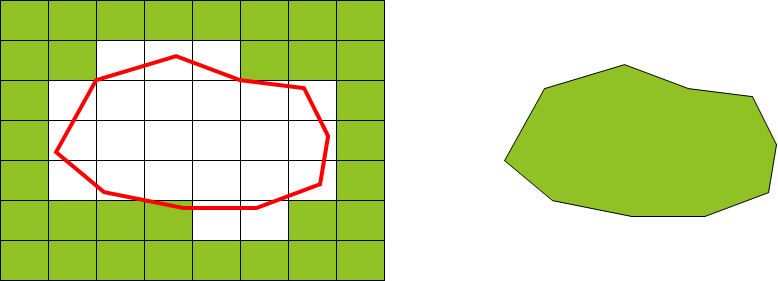### 8.2   Issues with resolution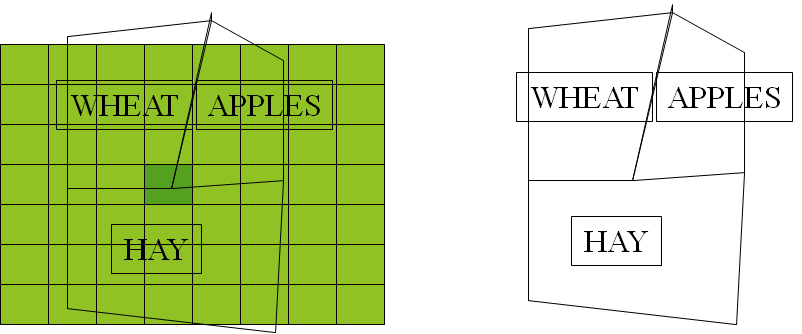## 9   Raster vs. vector

What is the best format to represent geographic objects in a GIS, raster or vector?

### 9.1   Shoreline example

In this example, the vector data source representing the shoreline appears to have greater detail and possibly greater accuracy.

### 9.2   When to use vector or raster?

Vector data can often store information in a more compact format than raster data and also work well with linear objects such as stream networks. Vector data is not suitable for representing terrain surfaces.

Raster data models are much better at representing information that is continuous in nature such as temperature where the value of temperature may be different between neighbors. Raster data is suitable for representing terrain surfaces.

### 9.3   Vector: Advantages and disadvantages

Advantages

• Good representation of reality
• Compact data structure
• Topology can be described in a network
• Accurate graphics

Disadvantages

• Complex data structure
• Simulation may be difficult
• Some spatial analysis is difficult or impossible to perform

### 9.4   Raster: Advantages and disadvantages

Advantages

• Simple data structure
• Easy overlay
• Various kinds of spatial analysis
• Uniform size and shape
• Cheaper technology

Disadvantages

• Large amount of data
• Less “pretty”
• Projection transformation is difficult
• Different scales between layers can be a nightmare
• May lose information due to generalization

## 10   Triangulated irregular network (TIN)

A triangulated irregular network (TIN) is a data model that is used to represent three-dimensional objects.

While the TIN model is somewhat more complex than the simple point, line, and polygon vector models, or the raster model, it is actually quite useful for representing elevations.

### 10.1   Issues with TIN

Can be too heavy and slow!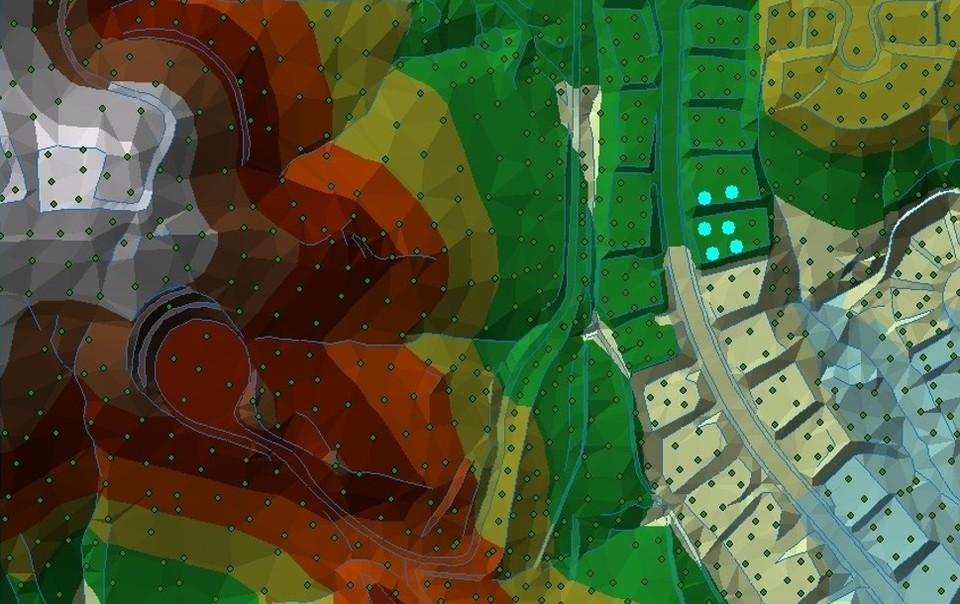## 11   Terrain dataset

Multi-resolution TIN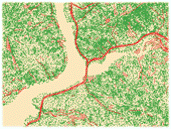Source points and breaklines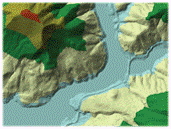High-resolution terrain dataset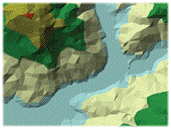Low-resolution terrain dataset

## 12   Common GIS file formats

### 12.1   Shapefile

Primary vector data file format used by many GIS programs

Consists of

• Shape file (*.shp): geometries
• Shape index (*.shx): shape indices
• Database table (*.dbf): attributes

Optionally

• Projection file (*.prj): projection information
• Ancillary files (*.sbn, *.sbx, etc.): spatial indices and other information used by ArcGIS
• Metadata files (*.htm, *.xml, *.txt): metadata

#### 12.1.1   Shape index

Stores the following two fields

• Record offset
• Record length

Makes reading geometries from a shapefile efficient and faster

#### 12.1.2   Spatial index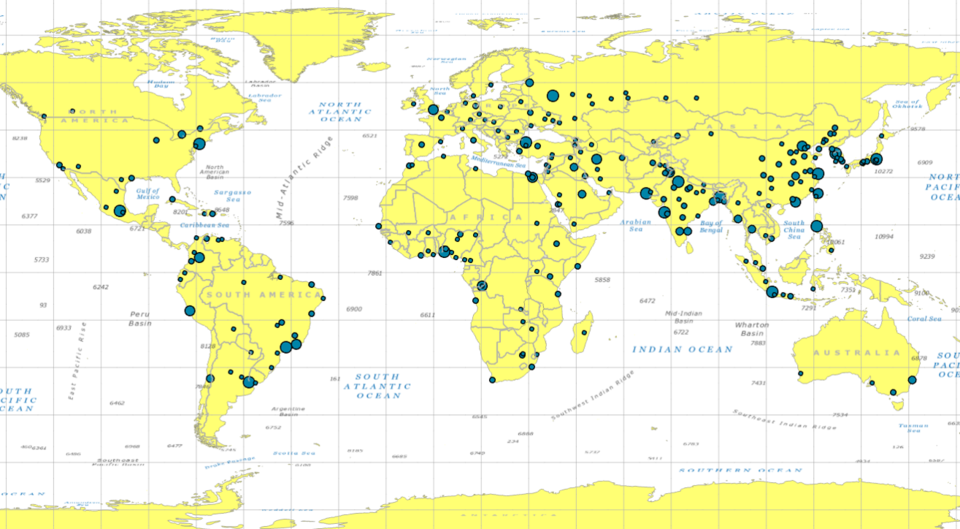### 12.2   GeoTiff

Primary raster data file format used by many GIS programs

*.tif or *.tiff

Yes, it’s an image file format with some geospatial metadata embedded, such as projections, extents, resolutions, etc.

## 13   Exercise: State plane and Universal Transverse Mercator (UTM) projections

Keywords: coordinate systems

In this exercise, we will import the polygons of state plane projections and UTM zones.